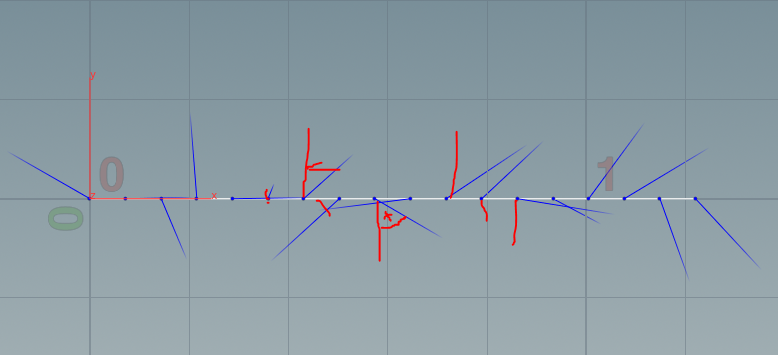## Recommended Posts

I need help I newly started with Houdini and doesn't know hot vops and vex works and I want to create a line where the normals are pointing in a different direction while keeping a 90-degree angle to the line. I fount a tutorial on the internet which only make the normals point in a random direction but not in the 90-degree angle I tried to set the normal direction to zero with floats and stuff but it always results in vex errors.

@N = sample_direction_uniform(rand(@ptnum));rand nomrals.hip##### Share on other sites

Hi,

There are many ways to do this. Here is one way:

```float r = radians ( 360 * rand ( @ptnum ) );
float x = sin ( r );
float z = cos ( r );

@N = set ( x, 0, z );```

##### Share on other sites
Posted (edited)
4 hours ago, animatrix said:

Hi,

There are many ways to do this. Here is one way:

```
float r = radians ( 360 * rand ( @ptnum ) );
float x = sin ( r );
float z = cos ( r );

@N = set ( x, 0, z );```float r = radians ( 360 * rand ( @ptnum ) );
float x = 0;
float z = 0;

@N = set ( 0, 1, r);

Thanks but the normals aren't random at a full 360 degrees and the normal size varies I need them no be uniform because I want them to drive the velocity of a pop simulation

edit: sorry my line was not on the right axis (wrong up vector) it works Thanks

Edited by efour

## Create an account

Register a new account